Arithmetic Operators (CNS) (Read 3557 times)

Started by Just No Point, June 06, 2014, 06:54:39 pm

Just No PointArithmetic Operators (CNS)
#1  June 06, 2014, 06:54:39 pm
••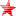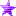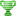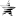Arithmetic operators allow you to perform basic operations like addition, multiplication, division, etc. MUGEN has a selection of operators that should be familiar to most programmers. They are as follows:

• +

Adds two numbers. If x and y are both ints, then x + y is also an int. If both are floats, then x + y is a float. If one is a float, then the other is promoted to float, and then the two floats are added.

• -

Subtraction of numbers. Type promotion works the same as addition.
• *

Multiplication of two numbers. Type promotion works the same as addition.
• /

Division of two numbers. If x and y are both ints, then x/y gives the integer quotient, i.e., the number of times that y goes evenly into x. For instance, 7/2 = 3. If x and y are both floats, then x/y returns a float. Our previous example would become 7.0/2.0 = 3.5. If only one of x or y is a float, then the other is promoted and float division is performed. Division by 0 will produce bottom.

• %

The remainder or mod operator. If x and y are both ints, then x % y gives the remainder when x is divided by y. If one or both are floats, or if y is 0, then bottom is produced.
• **

The exponentiation operator. If x and y are both non-negative ints, then x**y gives an int representing x raised to the power of y. (We define 0**0 = 1). However, it is very easy to overflow the maximum possible value for an int when computing large powers. In these cases MAX_INT (the largest possible integer) will be returned, and a warning will be generated. If one of x or y is negative or is a float, then both arguments are promoted to float and x**y is computed as an exponentiation of real numbers. An invalid exponentiation like -1 ** .5 will produce bottom.
• !

Logical NOT operator. !x evaluates to 0 (int) if x is nonzero, and 1 (int) if x is zero.
• &&

The logical AND operator. x && y evaluates to 1 (int) if x and y are both nonzero, and to 0 (int) otherwise.
• ||

The logical OR operator. x || y evaluates to 1 (int) if one or more of x and y is nonzero, and to 0 (int) otherwise.
• ^^

The logical XOR operator. x ^^ y evaluates to 1 (int) if exactly one of x and y is nonzero, and to 0 (int) otherwise.
• ~

The bitwise NOT operator. ~x inverts the bits of x's binary (two's complement) representation. Produces bottom if x is a float.

NOTE:This does not work as intended. Usage of this will result in a syntax error.
• &

The bitwise AND operator. The nth bit of x&y is set if and only if the nth bits of both x and y are set. Produces bottom if x or y is a float.
• |

The bitwise OR operator. The nth bit of x|y is set if and only if the nth bit of either x or of y (or both) is set. Returns bottom if either x or y is a float.
• ^

The bitwise XOR operator. The nth bit of x^y is set if and only if the nth bit of exactly one of x and y is set. Returns bottom if either of x or y is a float.
• =

The equality operator. If x and y are both ints or both floats, x = y evaluates to 1 (int) if x and y are equal, and 0 otherwise. If exactly one of x or y is a float, then they are both converted to floats before testing for equality.
• :=

The assignment operator. An unredirected variable name (var(n) or fvar(n) for suitable values of n) must appear on the left-hand side. If the left-hand side contains an integer variable, then the right-hand side is truncated to an integer before assignment. If the left-hand side contains a float variable, then the right-hand side is converted to float if necessary before assignment. In both cases, the value of the expression is the value that is assigned to the variable.
• !=

The inequality operator. If x and y are both ints or both floats, x != y evaluates to 1 (int) if x and y are not equal, and 0 otherwise. If exactly one of x or y is a float, then they are both converted to floats before testing for equality.
• <

The less-than operator. If x and y are both ints or both floats, x < y evaluates to 1 (int) if x < y, and 0 otherwise. If exactly one of x or y is a float, then they are both converted to floats before testing for equality.
• <=

Similar to <, with the exception that if x = y, then x <= y returns 1 (int).
• >

The greater-than operator. If x and y are both ints or both floats, x > y evaluates to 1 (int) if x > y, and 0 (int) otherwise. If exactly one of x or y is a float, then they are both converted to floats before testing for equality.
• >=

Similar to >, with the exception that if x = y, then x >= y returns 1 (int).
• =[,] !=[,] =[,) !=[,) =(,] !=(,] =(,) !=(,)

Interval operators. These take three arguments, x, y, and z. If any of x, y, or z is a float, they are all converted to floats. After conversion if necessary, x = [y,z] is equivalent to (x >= y) && (x <= z). Similarly, x = (y,z) is equivalent to (x > y) && (x < z). The half-open intervals have the obvious meaning.

The negated interval operators work as follows: x != [y,z] is equivalent (after conversion if necessary) to (x < y) || (x > z). x != (y,z) is equivalent to (x <= y) || (x >= z). The half-open intervals again have the obvious meaning.

You can view the interval operators as producing the appropriate open, closed, or half-open intervals on the ints or the floats. The = symbol means set membership in this context.

Some restrictions apply on where intervals may be placed in an expression. See the section on syntax for details.

Merged Operator specific question to this thread. Below is the question
Code:
trigger2 = var(14) % 50 = 1

Quote
%

The remainder or mod operator. If x and y are both ints, x%y returns an int representing the remainder after performing a division x/y. For instance, 7%2 = 1, and 23 % 17 = 6. It is an error to use the % operator on float values, or to compute x%0. The result of such operations will be discussed in the section on SC values.

This is from Rajaa's Necro. I'm studying how he made the config file and what all the pieces do. Right now I'm looking into his chaining coding.

What exactly does the % here do exactly? (Arkansas math was horrid when I was a kid, we didn't learn this crap)

Here is the full code it came from though I don't think you'll need it to explain
Spoiler: from CMD (click to see content)Last Edit: October 06, 2015, 11:21:40 pm by Just No Point

AlexSin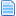Re: Arithmetic Operators (CNS)
#2  June 06, 2014, 06:58:24 pm
••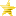From his example:
7/2 = 3, you get 1 as a remainder/rest.
23/17=1, you get 6 as the remainder/rest;

from the cmd:
var(14)/50 = you get a result that we don't care about in this instance, but you get the rest (it's 1, or 2, or 4, or 5).

so the % does the division and tells you the rest of the operation.

ByakkoRe: Arithmetic Operators (CNS)
#3  June 06, 2014, 07:02:27 pm
••Basically, for A%B=C, you take A, keep subtracting B until you can't anymore, and what's left is C. Var(40)%50=1 is true when var(40) has the value 1, 51, 101, 151 etc. C can't be a number higher than B.
If I struggled to the end of my determination, to the end of my way of life with my followers, if the result is ruin, then this ruin is inevitable. Grieve. Shed tears. But you cannot regret.

Just No PointRe: Arithmetic Operators (CNS)
#4  June 06, 2014, 07:05:24 pm
••Oh okay, I think I get it now! Won't know for sure till I apply it myself but that won't be today =p

Makes sense in my head though and as we've seen that's always a good sign! /sarcasmXGargoyleRe: Arithmetic Operators (CNS)
#5  June 06, 2014, 07:19:16 pm
••• Hedgehog Whisperer
• Red Bull addict
You will commonly use the mod operator for something that you want to trigger at a fixed and continuous interval, for example gametime%60=0 will trigger once per second, exactly on game tick 60, 120, 180, 240...

gametime%60=1 will trigger on tick 61, 121, 181, 241...

When using it in a variable, var(x)%10=0 will trigger every time var(x) increases or decreases 10 unitsXGargoyle: Battle posing since 1979http://xgargoyle.mgbr.nethttp://www.pandorabots.com/pandora/talk?botid=e71c0d43fe35093a  <-- Please click that linkhttp://paypal.me/XGargoyle  <-- Donations welcome!

Just No PointRe: Arithmetic Operators (CNS)
#6  October 06, 2015, 11:10:26 pm
••bump with new infoLast Edit: October 06, 2015, 11:21:21 pm by Just No Point

RicepigeonRe: Arithmetic Operators (CNS)
New #7  November 03, 2015, 09:43:20 pm
••• Gaps? Where we're going, we don't need gaps...
I know this was primarily covered in the Null Sctrl thread, but it should also be brought up here as well.

When using the assignment operator ( := ) in your triggers, be wary that variable assignments return the value of the assignment as their result, so a variable assignment of 0 will return False, thus the rest of your triggers may not activate. In such cases, it would be a good idea to include them in a logical OR statement to ensure that the rest of your triggers activate like so:

Code:
trigger1 = (var(0):=0)||1
trigger1 = (var(1):=0)||e

Additionally, you can use the assignment operator outside of triggers, including inside Statedefs like so:

Code:
[Statedef 200]
type = S
movetype = A
physics = S
anim = 200+0*(var(0):=0) ;at the beginning of the state, sets animation to animation number 200 AND sets var(0) to 0 simultaneously.

And another complex yet fun example;

Code:
[State 200, Changestate]
type = changestate
trigger1 = time = 10
trigger1 = cond(life<500,(var(1):=210),(var(1):=220))
value = var(1) ;At time=10, changes to State 210 if Life is less than 500, otherwise changes to state 220.

Or if you have multiple versions of the same special:

Code:
[state -1, Shoryuken]
type = changestate
value = 1100
triggerall = statetype != A
triggerall = ctrl || ((stateno=[200,499])&&movecontact)
trigger1 = command="623x"
trigger1 = (var(10):=1)||1
trigger2 = command="623y"
trigger2 = (var(10):=2)||1
trigger3 = command="623z"
trigger3 = (var(10):=3)||1

[Statedef 1100]
type = S
movetype = A
anim = 1100+1*(var(10)=2)+2*(var(10)=3) ;Changes animation based on button press
Last Edit: November 03, 2015, 09:52:58 pm by Ricepigeon